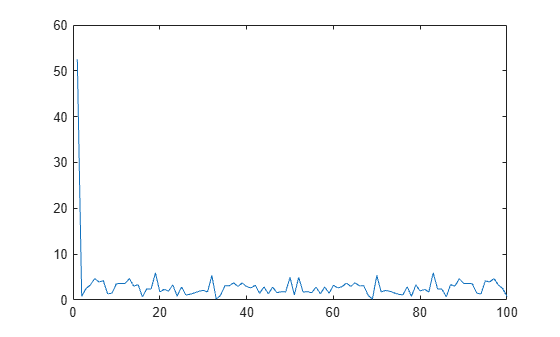This example shows how to create two different memory maps, and then read from each of the maps using the appropriate syntax. Then, it shows how to modify map properties and analyze your data.

You can read the contents of a file that you mapped to memory using the same MATLAB® commands you use to read variables from the MATLAB workspace. By accessing the Data property of the memory map, the contents of the mapped file appear as an array in the currently active workspace. To read the data you want from the file, simply index into the array. For better performance, copy the Data field to a variable, and then read the mapped file using this variable:

dataRef = m.Data;

for k = 1 : N

y(k) = dataRef(k);

end

By contrast, reading directly from the memmapfile object is slower:

for k = 1 : N

y(k) = m.Data(k);

end

Read from Memory Map as Numeric Array

First, create a sample data file named records.dat that contains a 5000-by-1 matrix of double-precision floating-point numbers.

rng('default')
randData = rand([5000,1]);

fileID = fopen('records.dat','w');
fwrite(fileID,randData,'double');
fclose(fileID);

Map 100 double-precision floating-point numbers from the file to memory, and then read a portion of the mapped data. Create the memory map, m. Specify an Offset value of 1024 to begin the map 1024 bytes from the start of the file. Specify a Repeat value of 100 to map 100 values.

m = memmapfile('records.dat','Format','double', ...
'Offset',1024,'Repeat',100);

Copy the Data property to a variable, d. Then, show the format of d.

d = m.Data;

whos d
Name        Size            Bytes  Class     Attributes

d         100x1               800  double

The mapped data is an 800-byte array because there are 100 double values, each requiring 8 bytes.

Read a selected set of numbers from the file by indexing into the vector, d.

d(15:20)
ans = 6×1

0.3510
0.5132
0.4018
0.0760
0.2399
0.1233

Read from Memory Map as Nonscalar Structure

Map portions of data in the file, records.dat, as a sequence of multiple data types.

Call the memmapfile function to create a memory map, m.

m = memmapfile('records.dat',  ...
'Format', {              ...
'uint16' [5 8] 'x';   ...
'double' [4 5] 'y' });

The Format parameter tells memmapfile to treat the first 80 bytes of the file as a 5-by-8 matrix of uint16 values, and the 160 bytes after that as a 4-by-5 matrix of double values. This pattern repeats until the end of the file is reached.

Copy the Data property to a variable, d.

d = m.Data
d=166×1 struct array with fields:
x
y

d is a 166-element structure array with two fields. d is a nonscalar structure array because the file is mapped as a repeating sequence of multiple data types.

Examine one structure in the array to show the format of each field.

d(3)
ans = struct with fields:
x: [5x8 uint16]
y: [4x5 double]

Read the x field of that structure from the file.

d(3).x
ans = 5x8 uint16 matrix

62645   30353   12492   16358   58958    9377   48754   16323
14152   21370   16352   21042   61010   33482   16321   22042
2657   16336   37389   35249   45699   16353   47136   59002
16360   41668    9638   33351   16366    3344   58286   31491
5368   55234   24278   16364   55768    7216    7184   16336

MATLAB formats the block of data as a 5-by-8 matrix of uint16 values, as specified by the Format property.

Read the y field of that structure from the file.

d(3).y
ans = 4×5

0.8407    0.9293    0.6160    0.5853    0.7572
0.2543    0.3500    0.4733    0.5497    0.7537
0.8143    0.1966    0.3517    0.9172    0.3804
0.2435    0.2511    0.8308    0.2858    0.5678

MATLAB formats the block of data as a 4-by-5 matrix of double values.

Modify Map Properties and Analyze Data

This part of the example shows how to plot the Fourier transform of data read from a file via a memory map. It then modifies several properties of the existing map, reads from a different part of the data file, and plots a histogram from that data.

Create a sample file named double.dat.

randData = rand([5000,1]);
fileID = fopen('double.dat','w');
fwrite(fileID,randData,'double');
fclose(fileID);

Create a memmapfile object of 1,000 elements of type double, starting at the 1025th byte.

m = memmapfile('double.dat','Offset',1024,  ...
'Format','double','Repeat',1000);

Copy the Data property to a variable, k. Then, get data associated with the map and plot the FFT of the first 100 values of the map.

k = m.Data;
plot(abs(fft(k(1:100))))This is the first time that data is referenced and is when the actual mapping of the file to the MATLAB address space takes place.

Change the map properties, but continue using the same file. Whenever you change the value of a memory map property, MATLAB remaps the file to memory.

m.Offset = 4096;
m.Format = 'single';
m.Repeat = 800;

m is now a memmapfile object of 800 elements of type single. The map now begins at the 4096th byte in the file, records.dat.

Read from the portion of the file that begins at the 4096th byte, and calculate the maximum value of the data. This command maps a new region and unmaps the previous region.

X = max(m.Data)
X = single
3.2278e+38